## Monday, 6 June 2011

### Calculate Day of Date in Salesforce

In Apex Class :

Way 1 :
Calculate using DateTime Format : It is very interesting way of finding the Day of a date.
```Date d = date.newinstance(1947,8, 15);
datetime myDate = datetime.newInstance(d.year(), d.month(), d.day());
String day = myDate.format('EEEE');

```

Way 2 : Prepared a class to find out day of given date using Mod of  7 to determine the day.

```public class DayOfDate {
Private Date startDate = date.newInstance(0001, 1, 1);
Public Date selectedDate{get;set;}
public String dayValue{get;set;}
public Account acc {get;set;}
public DayOfDate()
{
selectedDate = date.today();
acc = new Account();
}

public void calculateDayOfDate()
{
List<String> listDay = new List<String>{'Saturday' , 'Sunday' , 'Monday' , 'Tuesday' , 'Wednesday' , 'Thursday' , 'Friday'};
selectedDate = acc.SLAExpirationDate__c;
Integer remainder = Math.mod(startDate.daysBetween(selectedDate) , 7);
dayValue = listDay.get(remainder);
}

}
```

Visualforce Page :
```<apex:page controller="DayOfDate">
<apex:form>
<apex:pageBlock>

<apex:pageBlockSection columns="1">
<apex:pageBlockSectionItem>
<apex:outputLabel value="Enter Date"></apex:outputLabel>
<apex:inputField value="{!acc.SLAExpirationDate__c}"/>
</apex:pageBlockSectionItem>
<apex:pageBlockSectionItem>
<apex:outputLabel value="Day is : {!dayValue}"></apex:outputLabel>
</apex:pageBlockSectionItem>
<apex:commandButton action="{!calculateDayOfDate}" value="Find Day"/>

</apex:pageBlockSection>
</apex:pageBlock>
</apex:form>
</apex:page>
```

In above example I used Account objects date field  SLAExpirationDate__c to take input from user, you can take input using text field also, but in that case please format the date properly.

Calculate Day of a Date in formula field :

Create a formula field which should have return type 'Text'

```IF(MOD(DateFieldAPIName__c - DATE(0001,1,1) , 7) == 0 , 'Saturday' , IF(MOD(DateFieldAPIName__c- DATE(0001,1,1) , 7) == 1 , 'Sunday' ,
IF(MOD(DateFieldAPIName__c- DATE(0001,1,1) , 7) == 2 , 'Monday' ,
IF(MOD(DateFieldAPIName__c- DATE(0001,1,1) , 7) == 3 , 'Tuesday' ,
IF(MOD(DateFieldAPIName__c- DATE(0001,1,1) , 7) == 4 , 'Wednesday' ,
IF(MOD(DateFieldAPIName__c- DATE(0001,1,1) , 7) == 5 , 'Thursday' ,
IF(MOD(DateFieldAPIName__c- DATE(0001,1,1) , 7) == 6 , 'Friday' , 'No Day'))))))
)
```

In this formula DateFieldAPIName__c is the field api name of the date field for which you want to know the day of date.

You can also Use "Case" instead of  IF in in above formula like this :

```CASE(MOD(DateFieldAPIName__c - DATE(0001,1,1) , 7) , 0 , 'Saturday' , 1 , 'Sunday' ,  2 , 'Monday' , 3 , 'Tuesday' , 4 , 'Wednesday' , 5 , 'Thursday' , 6 , 'Friday'  , 'No Day Found')
```
Regards
Shashikant Sharma

#### 15 comments:

1.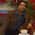Hi,

If we take a DateTime field we can get the Day in one line. See the below code, let me know if I am wrong.

DateTime currentTime = system.now();

system.debug('-------' + currentTime.format('EEEE'));

Run this code and you will get the Day :)

2.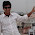@Gaurav
Yes, It worked fine :) , I have added this also in my post.

3.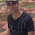Thanks Sir, I have implemented first one It is working fine really it is supportive approach.
Now My Question is what the "EEEE" is Stands for?

4.Hi Sandeep,

Symbol "E" stands for Day in week:
example
"EEE" -> "Tue"
"EEEE" -> "Tuesday"

This is basically referred form java.text.SimpleDateFormat class

The initialization is below: -
SimpleDateFormat format =
new SimpleDateFormat("EEE MMM dd HH:mm:ss zzz yyyy");

Let me know if you have any more queries.

Thanks,
Gaurav

5.@Gaurav thanks for this clarification ...

6.Figuring day of week helped me a lot! Thanks for above MOD formula!

7.thanks for posting this. the Case approach worked perfect!

8.This method will fail when using different user locales (i.e. the first day of the week is different for different Locale settings.

1.Yes your correct did you find any other approaches to do this?

9.Will doing the format('EEE') thing fail if the app is run by someone using another language?

10.11.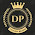hi,

I have a request which states that: i have one pick list field in which one value selects then the other date field to be populate to one day and if that populating day is saturday then it should populate 3 days and if it is sunday then 2 days.

please help me.

12.hi, i want to hide multiple controlling picklist fields... for example: i have A,B,C picklist fields and A is controlling B, B controlling C. Now i want to hide both A and B picklist fields in VF page. I know to hide One Controlling picklist field but i am unable to hide Two fields(in case A and B). Please suggest me.

13.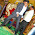I need help on this below.
If the selected date is saturday or sunday i need to show error.
Can any one help me on this

14.This comment has been removed by the author.